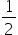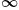Mathematics
Easy

Question

# Find the domain and range of f(x) = |2x - 1| + 3

## D : [,) , R : [3 ,)D : (-,) , R : (-,)D : (-,) , R : (3 ,)D : (,) . R : [-,)Hint:

## The correct answer is: D : (-,) , R : (3 ,)

### To find the domain and range of f(x) = |2x - 1| + 3

Hence, the domain of f(x) is the set of all real value of x and the range is [3,∞).

### Related Questions to study#### With Turito Foundation.#### Get an Expert Advice From Turito.